# P1 SCARA Project

I have just started working on this SCARA robot and find it very interesting.

I have all the stepper motors working and now I'm trying to figure out how many steps make a degree of movement. This is all about the math or geometry.

So the reach of the SCARA arm is about 200mm so the process is to determine how many steps equals 90 degrees for both the arm and the elbow.

Once I have that I can use the law of cosines to position the arm any where on the grid.

Mike

• Nice platform. Did you 3d print the parts yourself?
• I could have but it came as a kit with all the bearings and rods. Didn't want to mess with getting something wrong the first time. The belt on the arm is a little loose so they have an updated piece for that so I am printing that.

Mike
• That looks fun.
I hope you share a video once it's moving.
• @"Duane Degn",

Careful what you ask for. I got the angles working so I can control the Shoulder and Elbow by setting the angle. Now I need to correct the Elbow because the Shoulder messes with the Elbow angle. Something is connected here.

Here is a video with it in action using the Propeller Plug and Play board. SCARA on the move.

Mike
• iseries wrote: »
Here is a video with it in action

Very cool. Thanks.

I hope you keep adding videos as you make progress controlling it.

• +1
• My next issue was that because the pivot point where the Shoulder and Elbow are driven from cause the angle to change as the Shoulder moves I needed to correct for this in the Elbow.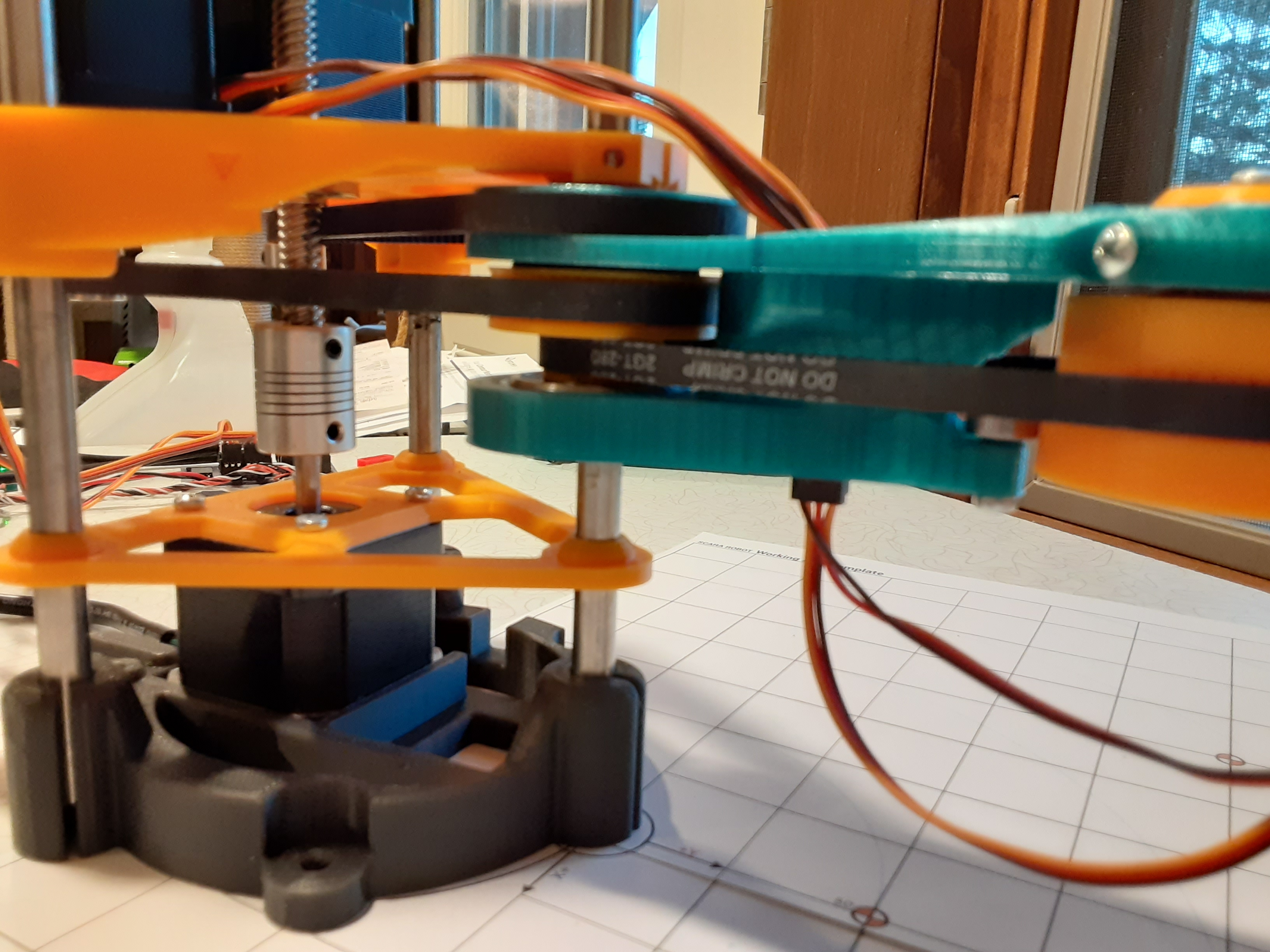So if you request that the Elbow is 90 degrees from the Shoulder and the Shoulder is 90 degrees as well that you should end up with Elbow pointing straight up.

Here is a video of the arm in action. You will note that because each stepper motor is in it's own cog they can all move together at once. Arm in Action

Mike
• Alright you trigonometry lovers I think this project qualifies as one. I need to calculate a number of angles to correctly position the arm.
I have a grid that is 200mm by 200my both in the positive and negative direction so it’s 400 mm all together.
The robot starts at 0 X and 200 Y with the arm pointing straight out. The Shoulder angle will be 0 degrees and the Elbow angle will also be 0 degrees at this point.
Now I need to position the robot at say 100 X and 100 Y. What angles do I need to generate for the Shoulder and Elbow to put the arm at the correct position assuming I have a left arm. The calculation is different if I have a right arm.
Here is a picture of the arm.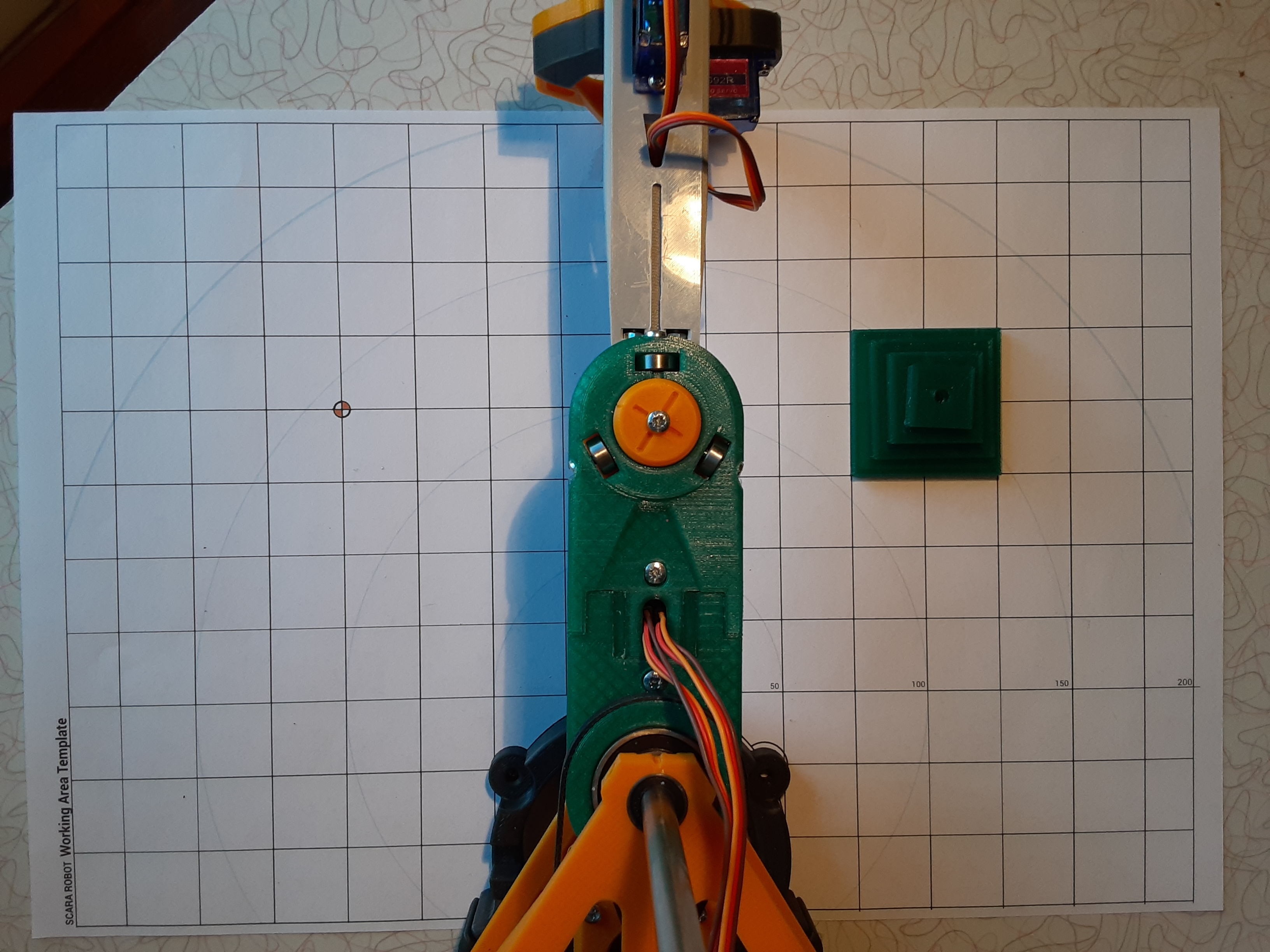The Shoulder arm length is 93mm and the Elbow arm is 105.92mm. Given this we can see that if we bend the Elbow at 90 degrees we would be at 93 X, 105.92 Y which is close to are end position of 100, 100.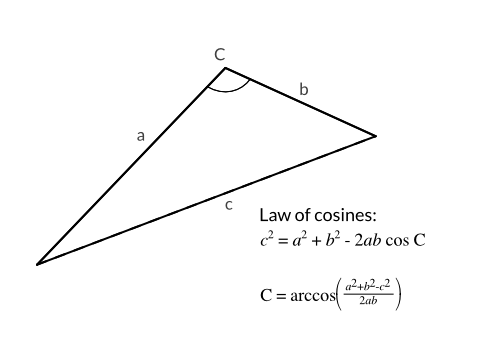Using the law of cosines lets calculate that angle. a=93, b=105.92, and c is what? Well we know that the end point is 100, 100 so are c length would be sqrt(x*x+y*y) or about 141. So the Elbow angle would be...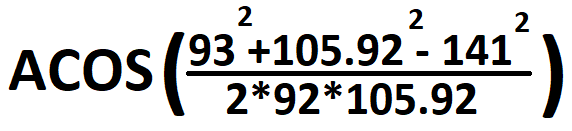That would be about 90 degrees which seems about right. Now we need the Shoulder angle which we could just use the sine function but will again use the Law of cosines this time using a and c.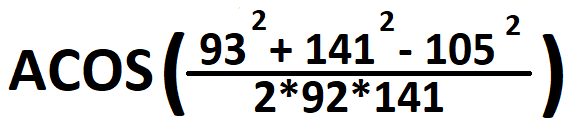That would be about 48.5 degrees. That angle has no reference to are point on the graph so we need to add to it the X, Y angle which is ATAN2(X,Y) or 45 degrees. That gives us an angle of 93 degrees which would be -3 degree for are left hand robot.
Instead of making these calculation on the P1 I used a C# program to do the calculations and then feed those angles to the P1 for processing. I also recorded those calculations so that I could play them back later one after another to move the pieces around on the grid.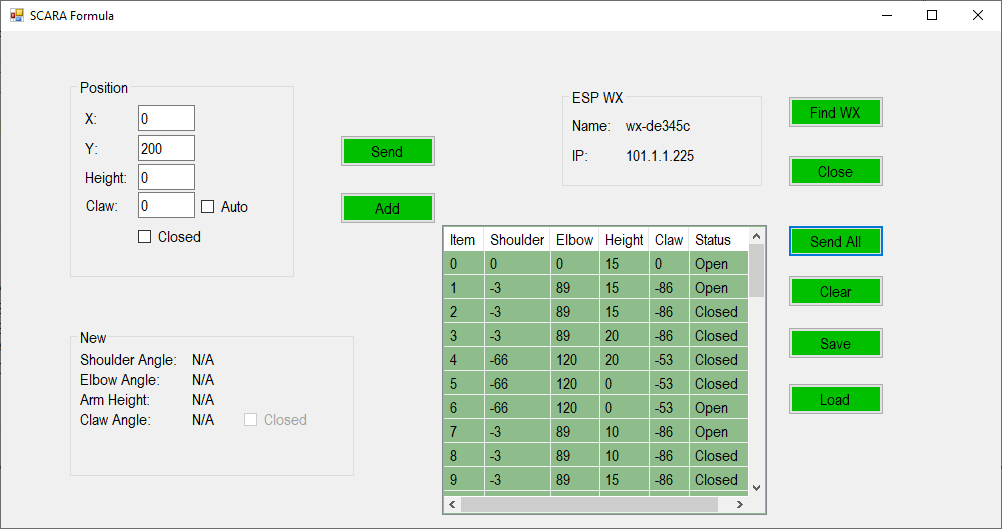Because I am using the WX module a simple UDP broadcast will return the IP address of it so that I can open a telnet connection to it. From there I send JSON packets with the 6 pieces of information needed to move the robot in sequence. [code}“{"Claw":-86,"Elbow":89,"Height":15,"Item":1,"OpenClose":0,"Shoulder":-3}”[/code]
Here is the robot in action.
SCARA Moving Pieces

I don't know if all this math is correct, I may have just been dreaming, but the robot does what I want it to do. Also you will note that the Claw stays at 90 degrees so that it is always square to the piece it's going to pick up. This involved more head scratching to come up with that angle as well. Now I need to figure out how to do right hand calculation so that the arm can get to negative locations beyond the reach of the left hand.

Later I may push all the math onto the P1 since I have it mostly debugged now.

Mike
• The robot looks good in the videoI noticed that the blocks appear to not be perfectly aligned (square to the grid). I. Suspect you will have to calculate another angle for the hand to square the jaws up. It’s too early, and no coffee, to look at your maths. I use Pythagorean’s theorem often in pcb layouts.